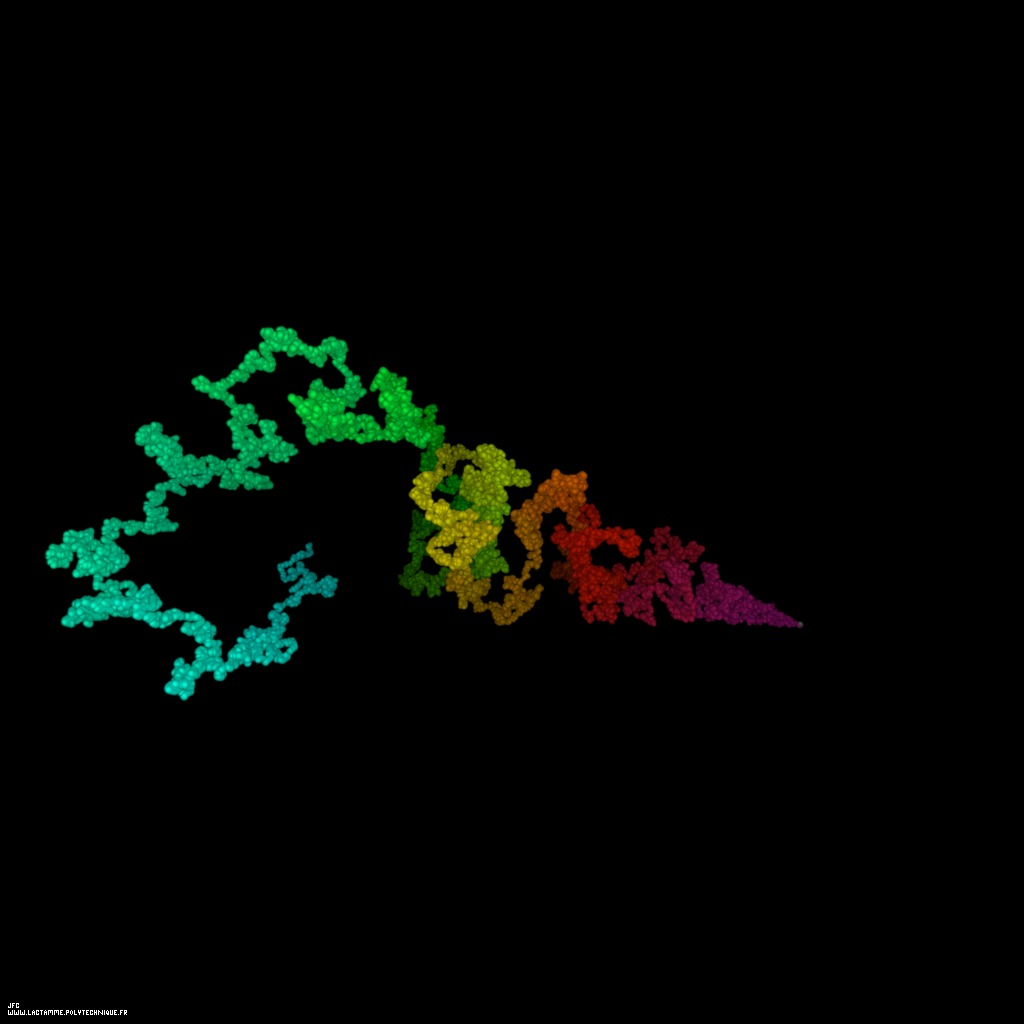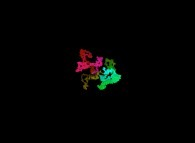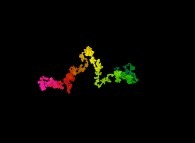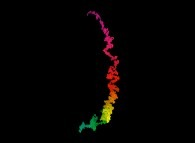The Liouville function displayed as a tridimensional random walk for the integer numbers from 2 to 150001 [La fonction de Liouville visualisée comme une marche aléatoire tridimensionnelle pour les nombres entiers de 2 à 150001].

This picture displays the Liouville function as a tridimensional random walk. For each integer number 'n' the function PD(n) gives the number of prime divisors; for example:
```                    PD(2) = 1
PD(3) = 1
PD(4) = 2
PD(5) = 1
PD(6) = 2
PD(7) = 1
PD(8) = 3
PD(9) = 2
(...)
```
Let's recall that 1 is not a prime number when 2 is the first one (and the only even one...).

The Liouville function L(n) equals:
```                               PD(n)
L(n) = (-1)
```
Hence:
```                    L(2) = -1
L(3) = -1
L(4) = +1
L(5) = -1
L(6) = +1
L(7) = -1
L(8) = -1
L(9) = +1
(...)
```

Then one defines the following dynamics:
```                    X(0) = 0
Y(0) = 0
Z(0) = 0
```
```                    X(i+1) = X(i) + L(3*i+2)
Y(i+1) = Y(i) + L(3*i+3)
Z(i+1) = Z(i) + L(3*i+4)
```
(the point {X(0),Y(0),Z(0)} is at the right of the picture -white point-, when the colors used {magenta,red,yellow,green,cyan} are an increasing function of 'i')

It is useful to compare this trajectorywith the one of a tridimensional brownian motion based on a random processand the one of a tridimensional brownian motion based on the Verhulst dynamics.

At last, here is the special Liouville functionwhere only the different prime divisors are counted.

(CMAP28 WWW site: this page was created on 01/07/2010 and last updated on 04/26/2015 12:24:47 -CEST-)

[See all related pictures (including this one) [Voir toutes les images associées (incluant celle-ci)]]

[Please visit the related NumberTheory picture gallery [Visitez la galerie d'images NumberTheory associée]]
[Go back to AVirtualMachineForExploringSpaceTimeAndBeyond [Retour à AVirtualMachineForExploringSpaceTimeAndBeyond]]
[The Y2K bug [Le bug de l'an 2000]]

[Site Map, Help and Search [Plan du Site, Aide et Recherche]]
[Mail [Courrier]]
[About Pictures and Animations [A Propos des Images et des Animations]]